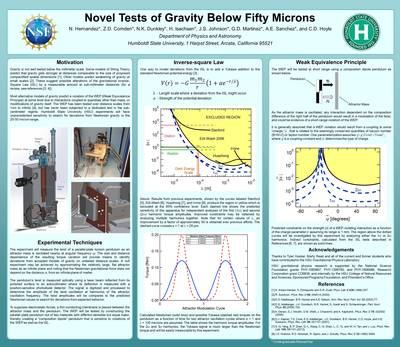INRSEP Research Posters

PreviewFall 10-2017

Abstract

Gravity’s relationship to the other fundamental forces is still not well understood. The Standard Model of quantum mechanics describes interactions between the strong, weak and electromagnetic forces, while Einstein’s theory of General Relativity (GR) describes gravity. There is yet to be a theory that unifies inconsistencies between GR and quantum mechanics. Scenarios of String Theory predicting more than three spatial dimensions also predict physical effects of gravity at sub-millimeter levels that could alter the gravitational inverse-square law. The Weak Equivalence Principle (WEP), a central feature of GR, states that all objects are accelerated at the same rate in a gravitational field independent of their composition. A violation of the WEP at any distance scale would be evidence that current models of gravity are incorrect. At the Humboldt State University Gravitational Research Laboratory, an experiment is being developed to observe gravitational interactions below the 50-micron distance scale. The experiment measures the twist of a parallel-plate torsion pendulum as an attractor mass is oscillated within 100 microns of the pendulum, providing time varying gravitational torque on the pendulum. The size and distance dependence of the torque amplitude provide means to determine deviations from accepted models of gravity on untested distance scales.

1

APS Far West

COinS